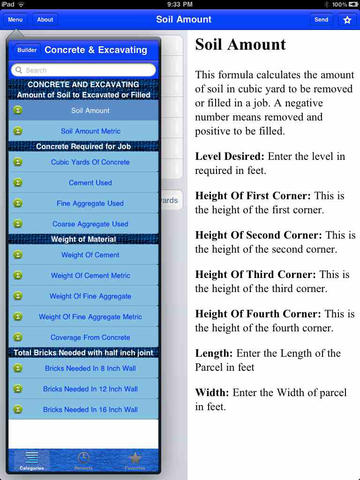Version: 3.1
Size: 61.6 MB
\$9.99

## Description

```Weekend Sale
The BUILDER'S FORMULATOR is the perfect tool for any general contractor or builder. This program contains over 200 formulas helpful for builders. BUILDER'S FORMULATOR combines all of the formulas from our Electrical, Carpentry and Plumbing Formulators. In addition, this product also includes Concrete and Excavations formulas, that perform calculations for excavating or filling soil, the concrete required for a job and the bricks required for a wall.

The CARPENTRY section contains formulates that calculate the number of joists and studs required on a job. BUILDER'S FORMULATOR aids users to calculate the load on headers or the cost of hardwood flooring. There are even formulas to calculate the loads on walls and loads on beams.

Formulas in the ELECTRICAL section calculate kilowatts, horsepower, efficiency, power factor, watts, amps, current, power, resistance, and voltage. This section includes formulas to calculate total resistance, capacitance, voltage drops, and transformer calculations.

The formulas in the PLUMBING section calculate areas of pipe walls and the weights of different pipes. BUILDER'S FORMULATOR is able to calculate the final temperature when two bodies of water are mixed. There are also formulas in this section to calculate the expansion of pipes, radiant heat and heat loss cooling requirments, head loss and fresh air in a room requirements.

Finally, BUILDER'S FORMULATOR includes a FINANCIAL section with formulas that calculate mortgages and even calculate price quotes. All formulas can be saved, and your can access recent or favorite formulas.```

## What's New in Version 3.1

`iPhone 5 Compatible, iPad Retina compatible, dozens of additional formulas`

## Screenshots for для Айфона, Айпада